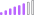cancel
Showing results for
Did you mean:Post Patron

## Calculating percentage of sum of revenue of each month in total revenue

Hello,

I have the following data:I would like to know how much percentage january is of the total sales.

1 ACCEPTED SOLUTIONSuper User

@Niels_T , Assume you have revenue measure =

Divide([Revenue], calculate([Revenue], allselected()) )

or

by yearSuper User

@Niels_T , Assume you have revenue measure =

Divide([Revenue], calculate([Revenue], allselected()) )

or

by year